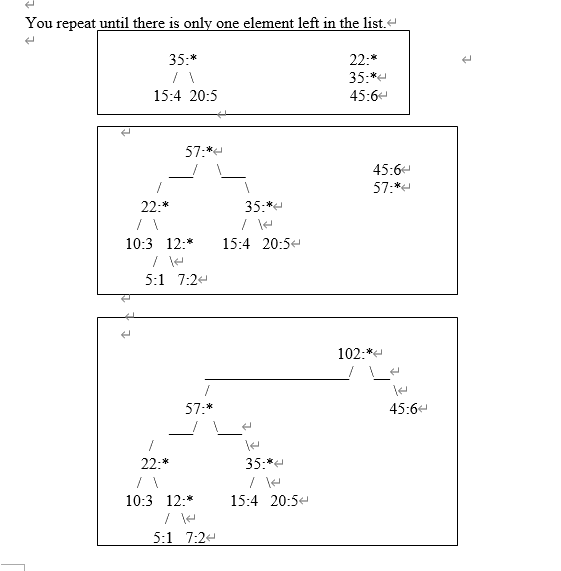2021-06-22 21:40

# 数据结构问题，需要直接答案。In this assignment, first you read a text file as input and obtain the frequencies (count) of each character in a given text file, including the blank spaces. Next, using the frequency information you obtained from the file you build a Huffman tree.

1. By tracing the branches of the Huffman tree your program outputs each character and the corresponding binary string.
2. Challenge (100 pts bonus):
1. Your program reopens the text file and encode each character as you read from the file using the Huffman tree you constructed in (a). The encoded file should appear in a file called encoded.txt in the same directory as the original file. Recall that each character you read cannot have a value greater than 255.
2. Using the encoded.txt binary file, your program decodes the encoded file and outputs the text data in decode.txt file. This file should be exactly the same as the original file.

Here is a brief discussion of Huffman encoding. Consider set of numbers and their frequency of use and want to create a Huffman encoding for them:

FREQUENCY   VALUE

5                              1

7                              2

10                             3

15                             4

20                             5

45                             6

Creating a Huffman tree is simple. Sort this list by frequency and make the two-lowest elements into leaves, creating a parent node with a frequency that is the sum of the two lower element's frequencies:

12:*

/  \

5:1   7:2

The two elements are removed from the list and the new parent node, with frequency 12, is inserted into the list by frequency. So now the list, sorted by frequency, is:

10:3

12:*

15:4

20:5

45:6

You then repeat the loop, combining the two lowest elements.

This results in:

22:*

/   \

10:3   12:*

/   \

5:1   7:2

and the list is now:

20:5

22:*

45:6

You repeat until there is only one element left in the list.（放图片里了，格式有问题）Now the list is just one element containing 102:*, you are done. This element becomes the root of your binary Huffman tree.

To generate a Huffman code you traverse the tree to the value you want, outputting a 0 every time you take a left hand branch, and a 1 every time you take a right hand branch. (normally you traverse the tree backwards from the code you want and build the binary huffman encoding string backwards as well, since the first bit must start from the top).

• 点赞
• 写回答
• 关注问题
• 收藏
• 邀请回答

#### 1条回答默认 最新

•王大师王文峰 2021-06-23 17:40
已采纳

望采纳，谢谢

``````#include <iostream>
#include <stdlib.h>
using namespace std;
const int MaxValue = 10000;//初始设定的权值最大值
const int MaxBit = 4;//初始设定的最大编码位数
const int MaxN = 10;//初始设定的最大结点个数
struct HaffNode//哈夫曼树的结点结构
{
int weight;//权值
int flag;//标记
int parent;//双亲结点下标
int leftChild;//左孩子下标
int rightChild;//右孩子下标
};
struct Code//存放哈夫曼编码的数据元素结构
{
int bit[MaxBit];//数组
int start;//编码的起始下标
int weight;//字符的权值
};
void Haffman(int weight[], int n, HaffNode haffTree[])
//建立叶结点个数为n权值为weight的哈夫曼树haffTree
{
int j, m1, m2, x1, x2;
//哈夫曼树haffTree初始化。n个叶结点的哈夫曼树共有2n-1个结点
for (int i = 0; i<2 * n - 1; i++)
{
if (i<n)
haffTree[i].weight = weight[i];
else
haffTree[i].weight = 0;
//注意这里没打else那{}，故无论是n个叶子节点还是n-1个非叶子节点都会进行下面4步的初始化
haffTree[i].parent = 0;
haffTree[i].flag = 0;
haffTree[i].leftChild = -1;
haffTree[i].rightChild = -1;
}
//构造哈夫曼树haffTree的n-1个非叶结点
for (int i = 0; i<n - 1; i++)
{
m1 = m2 = MaxValue;//Maxvalue=10000;(就是一个相当大的数)
x1 = x2 = 0;//x1、x2是用来保存最小的两个值在数组对应的下标

for (j = 0; j<n + i; j++)//循环找出所有权重中，最小的二个值--morgan
{
if (haffTree[j].weight<m1&&haffTree[j].flag == 0)
{
m2 = m1;
x2 = x1;
m1 = haffTree[j].weight;
x1 = j;
}
else if(haffTree[j].weight<m2&&haffTree[j].flag == 0)
{
m2 = haffTree[j].weight;
x2 = j;
}
}
//将找出的两棵权值最小的子树合并为一棵子树
haffTree[x1].parent = n + i;
haffTree[x2].parent = n + i;
haffTree[x1].flag = 1;
haffTree[x2].flag = 1;
haffTree[n + i].weight = haffTree[x1].weight + haffTree[x2].weight;
haffTree[n + i].leftChild = x1;
haffTree[n + i].rightChild = x2;
}
}
void HaffmanCode(HaffNode haffTree[], int n, Code haffCode[])
//由n个结点的哈夫曼树haffTree构造哈夫曼编码haffCode
{
Code *cd = new Code;
int child, parent;
//求n个叶结点的哈夫曼编码
for (int i = 0; i<n; i++)
{
//cd->start=n-1;//不等长编码的最后一位为n-1,
cd->start = 0;//,----修改从0开始计数--morgan
cd->weight = haffTree[i].weight;//取得编码对应权值的字符
child = i;
parent = haffTree[child].parent;
//由叶结点向上直到根结点
while (parent != 0)
{
if (haffTree[parent].leftChild == child)
cd->bit[cd->start] = 0;//左孩子结点编码0
else
cd->bit[cd->start] = 1;//右孩子结点编码1
//cd->start--;
cd->start++;//改成编码自增--morgan
child = parent;
parent = haffTree[child].parent;
}
//保存叶结点的编码和不等长编码的起始位
//for(intj=cd->start+1;j<n;j++)
for (int j = cd->start - 1; j >= 0; j--)//重新修改编码，从根节点开始计数--morgan
haffCode[i].bit[cd->start - j - 1] = cd->bit[j];

haffCode[i].start = cd->start;
haffCode[i].weight = cd->weight;//保存编码对应的权值
}
}
int main()
{
int i, j, n = 4, m = 0;
int weight[] = { 2,4,5,7 };
HaffNode*myHaffTree = new HaffNode[2 * n - 1];
Code*myHaffCode = new Code[n];
if (n>MaxN)
{
cout << "定义的n越界，修改MaxN!" << endl;
exit(0);
}
Haffman(weight, n, myHaffTree);
HaffmanCode(myHaffTree, n, myHaffCode);
//输出每个叶结点的哈夫曼编码
for (i = 0; i<n; i++)
{
cout << "Weight=" << myHaffCode[i].weight << "  Code=";
//for(j=myHaffCode[i].start+1;j<n;j++)
for (j = 0; j<myHaffCode[i].start; j++)
cout << myHaffCode[i].bit[j];
m = m + myHaffCode[i].weight*myHaffCode[i].start;
cout << endl;
}
cout << "huffman's WPL is:";
cout << m;
cout << endl;
return 0;
}``````
点赞 打赏 评论